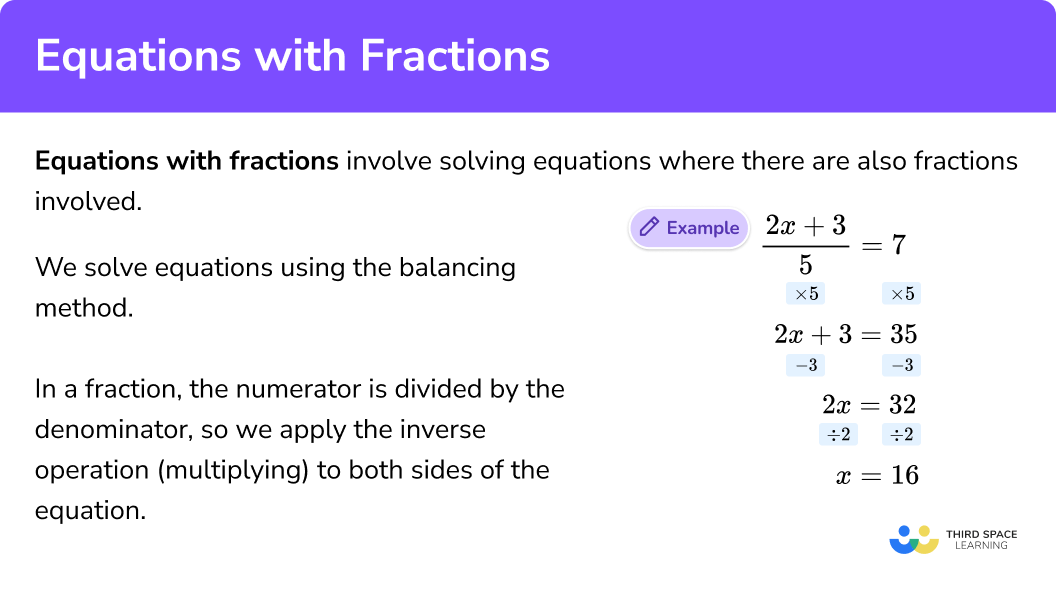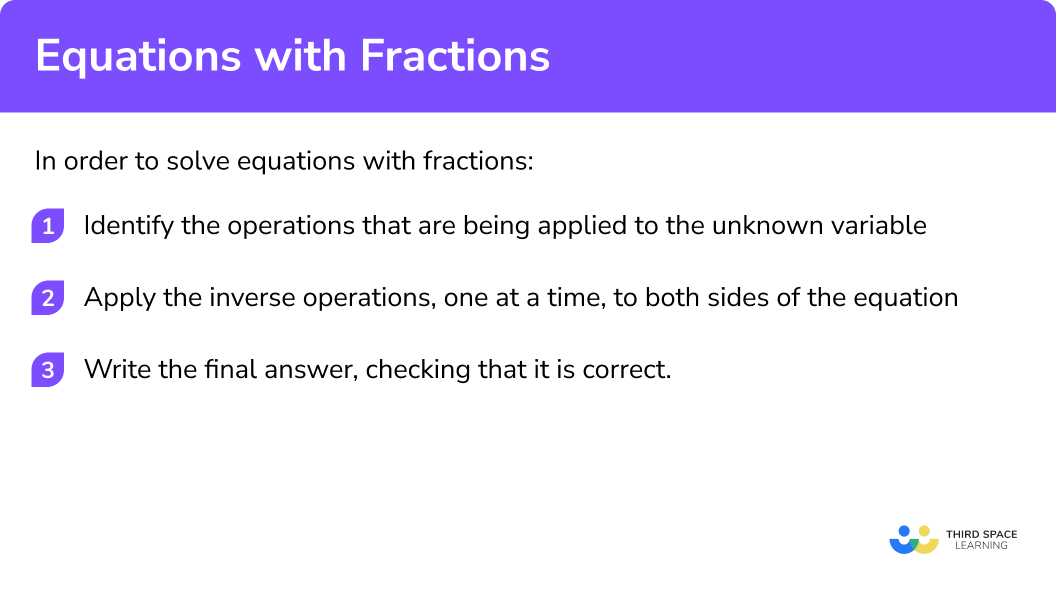GCSE Maths Algebra Equations Solving Equations

Equations With Fractions

# Equations With Fractions

Here we will learn about equations with fractions, including solving equations with fractions where the unknown is the denominator of a fraction.

There are also equations with fractions worksheets based on Edexcel, AQA and OCR exam questions, along with further guidance on where to go next if you’re still stuck.

## What are equations with fractions?

Equations with fractions involve solving equations where the unknown variable is part of the numerator and/or the denominator of the fraction.

To solve equations with fractions we need to work out what the value of the unknown variable. We solve equations by using the “balancing method” by applying the inverse operation to both sides of the equation.

The inverse operation of addition is subtraction.

The inverse operation of subtraction is addition.

The inverse operation of multiplication is division.

The inverse operation of division is multiplication.

For example,

### What are equations with fractions?## How to solve equations with fractions

In order to solve equations with fraction:

1. Identify the operations that are being applied to the unknown variable.
2. Apply the inverse operations, one at a time, to both sides of the equation.
3. Write the final answer, checking that it is correct.

### How to solve equations with fractions### Related lessons on solving equations

Equations with fractions is part of our series of lessons to support revision on solving equations. You may find it helpful to start with the main solving equations lesson for a summary of what to expect, or use the step by step guides below for further detail on individual topics. Other lessons in this series include:

## Equations with fractions examples

### Example 1: equations with one operation

Solve:

$\frac{x}{5}=4$

1. Identify the operations that are being applied to the unknown variable.

The unknown variable is x
Looking at the left hand side of the equation, the x is divided by 5 (the denominator of the fraction).

$\frac{x}{5}$

2Apply the inverse operations, one at a time, to both sides of the equation.

The inverse of “dividing by 5” is “multiplying by 5”.
We need to multiply both sides of the equation by 5.

3Write the final answer, checking that it is correct.

$x=20$

We can check the answer by substituting the answer back into the original equation.

$\frac{20}{5}=20\div5=4$

### Example 2: equations with one operation

Solve:

$\frac{x}{3}=8$

Identify the operations that are being applied to the unknown variable.

Apply the inverse operations, one at a time, to both sides of the equation.

Write the final answer, checking that it is correct.

### Example 3: equations with two operations

Solve:

$\frac{x+1}{2}=7$

Identify the operations that are being applied to the unknown variable.

Apply the inverse operations, one at a time, to both sides of the equation.

Write the final answer, checking that it is correct.

### Example 4: equations with two operations

Solve:

$\frac{x}{4}-2=3$

Identify the operations that are being applied to the unknown variable.

Apply the inverse operations, one at a time, to both sides of the equation.

Write the final answer, checking that it is correct.

### Example 5: equations with three operations

Solve:

$\frac{3x}{5}+1=7$

Identify the operations that are being applied to the unknown variable.

Apply the inverse operations, one at a time, to both sides of the equation.

Write the final answer, checking that it is correct.

### Example 6: equations with three operations

Solve:

$\frac{2x-1}{7}=3$

Identify the operations that are being applied to the unknown variable.

Apply the inverse operations, one at a time, to both sides of the equation.

Write the final answer, checking that it is correct.

### Example 7: equations with the unknown as the denominator

Solve:

$\frac{24}{x}=6$

Identify the operations that are being applied to the unknown variable.

Apply the inverse operations, one at a time, to both sides of the equation.

Write the final answer, checking that it is correct.

### Example 8: equations with the unknown as the denominator

Solve:

$\frac{18}{x}-6=3$

Identify the operations that are being applied to the unknown variable.

Apply the inverse operations, one at a time, to both sides of the equation.

Write the final answer, checking that it is correct.

### Common misconceptions

• Types of number

The solution to an equation can be different types of number. The unknown does not have to be an integer (whole numbers), it can also be a fraction or a decimal and can be positive or negative.

• The side of the equation that th unknown is on

The unknown variable, represented by a letter, is often on the left hand side of the equations however it doesn’t have to be. It could also be on the right hand side of an equation.

• Multiplying both sides of an equation

When multiplying each side of the equation of a number, it is a common mistake to forget to multiply every term.
E.g.
Solve: \frac{x}{2}+3=9

Here we have not multiplied the +3 by 2 resulting in the incorrect answer:

Here we have correctly multiplied each term by the denominator:

• Lowest common denominator (LCD)

It is common to get confused between solving equations involving fractions and adding and subtracting fractions. When adding and subtracting we need to work out the lowest/least common denominator (sometimes called the lowest common multiple or lcm) whereas when we solve equations involving fractions we need to multiply both sides of the equation by the denominator of the fraction.

### Practice equations with fractions questions

1. Solve: \frac{x}{6}=3

x=18x=9x=36x=122. Solve: \frac{x+4}{2}=7

x=18x=10x=26x=303. Solve: \frac{x}{8}-5=1

x=40x=48x=64x=564. Solve: \frac{3x+2}{4}=2

x=4x=16x=12x=25. Solve: \frac{4x}{7}-2=6

x=11x=7x=14x=106. Solve: \frac{42}{x}=7

x=5x=294x=7x=6### Equations with fractions GCSE questions

1. Solve \frac{x}{5}=3

(1 mark)

x=15

(1)

2. Solve \frac{x-3}{7}=2

(2 marks)

x-3=14

for the correct first step

(1)

x=17

(1)

3. Solve \frac{5a+6}{2}=23

(3 marks)

5a+6=46
for the correct first step

(1)

5a=40
for the correct second step

(1)

x=8

(1)

## Learning checklist

You have now learned how to:

• Solve equations when there are fractions
• Solve fractions where the unknown is the denominator

## Still stuck?

Prepare your KS4 students for maths GCSEs success with Third Space Learning. Weekly online one to one GCSE maths revision lessons delivered by expert maths tutors.

Find out more about our GCSE maths tuition programme.